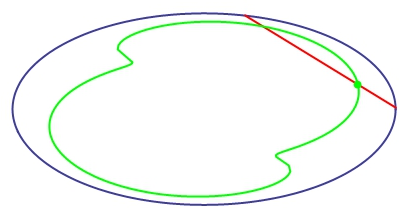# Pool Math

Calculus Level 4In a pool party, you decide to test your mad Math skills.

Having nothing better to do, you place a rigid iron bar inside a section c of an empty pool such that either end of it lies on the same plane and touch the insides of the pool. You then choose a point P on the bar such that the distance to one end is 7 and the distance to the other end is 3, with the length of the bar being 10. Finally, you move the bar in a way that, at any given time, neither end leaves c.

Suppose that each pool is a surface such that its horizontal sections are convex closed curves.

After one lap - at the moment the bar is back where it was first placed - what is the area between the curve covered by point P and the border of c, rounded to the closest integer?

×

Problem Loading...

Note Loading...

Set Loading...